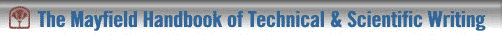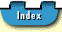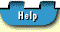## Bar Graphs

Bar graphs display relationships among data by means of vertical or horizontal bars of different different lengths. Sometimes a single bar of a set height is used to break down percentages of the whole, in much the same fashion as a pie graph.

#### Single Bar Graph

Single bar graphs visualize the effects of varying limiting conditions on one particular object of study, as in Figure 5, where heart rate is shown under various burden conditions of exercise.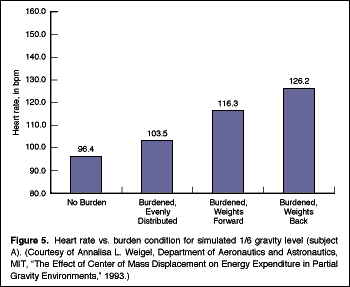#### Multiple Bar Graph

Multiple bar graphs visualize the different objects of study under one particular limiting condition, as in Figure 6, where three items are compared over time.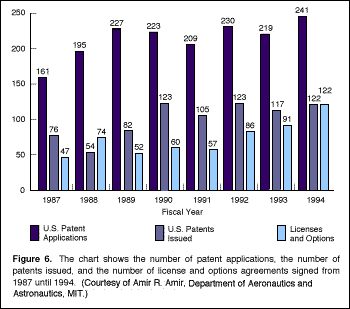#### Stacked Bar Graph

Stacked bar graphs visualize various items as percentages of the whole for ease of comparison and contrast. Figure 7 is a single bar graph showing percentages of one particular object of study. (Compare this figure with Figure 11, a pie graph drawn from the same data.) Figure 2 is an example of a multiple stacked bar graph where various items are broken down into percentages of the whole for comparison.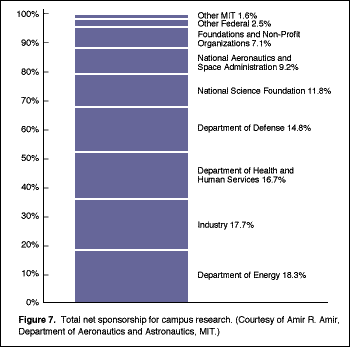## Bar Graphs ##